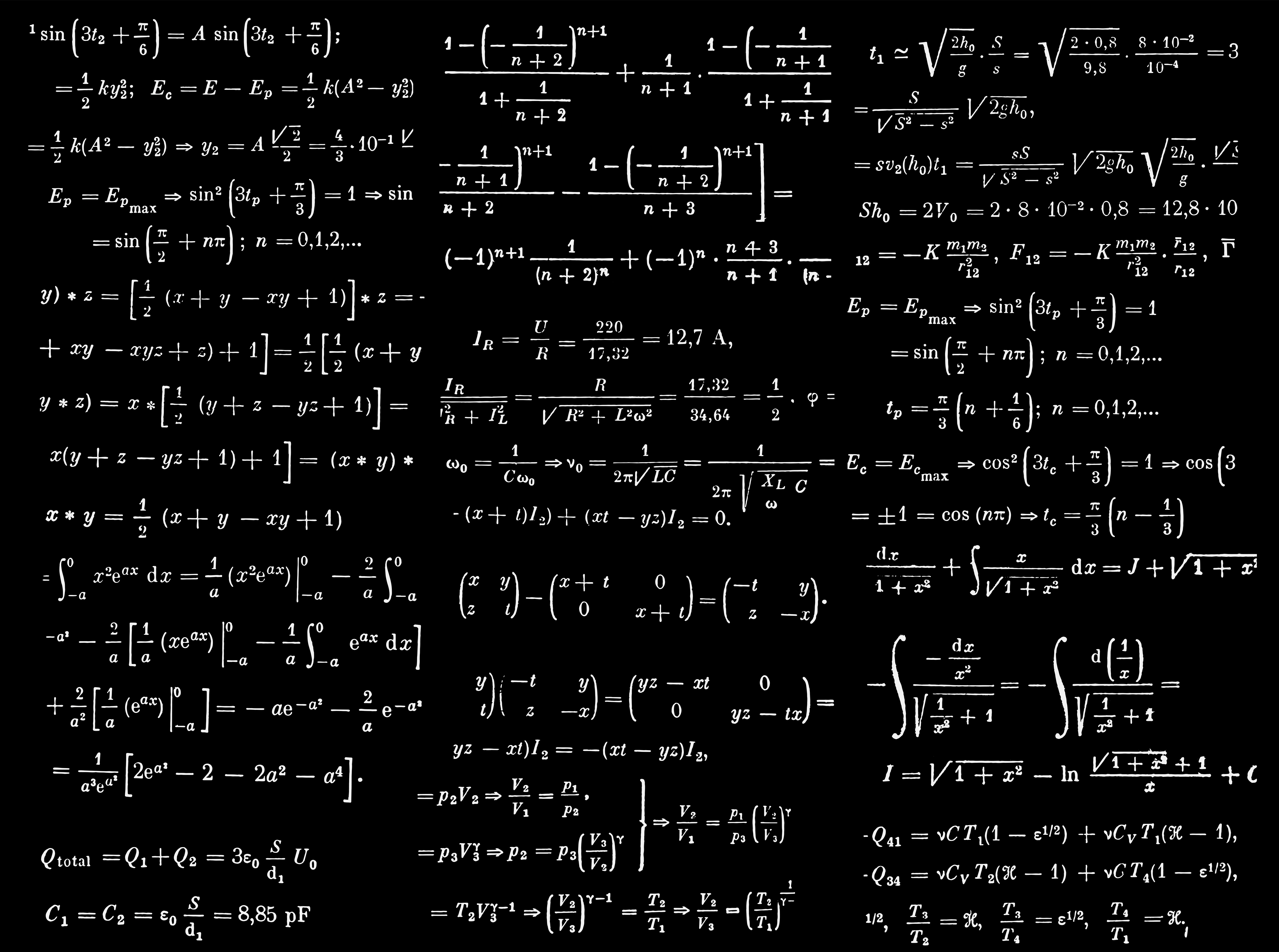# Introduction to Using Formulas in Notion for Automated Calculations

2 min read The Notion Nook

Notion is a productivity tool that allows users to create custom databases, organize their tasks and projects, and collaborate with others. One of the most useful features of Notion is the ability to use formulas to automate calculations and data analysis within their databases.

Formulas in Notion are written using a combination of functions and operators, and they are applied to columns in a database. To create a formula, you first need to create a database by clicking the “+” icon in the sidebar and selecting “Database” from the menu. Once you have created your database, click on the column where you want the result of the formula to appear, and then select “Formula” from the column type menu. This will open the formula editor, where you can enter the formula that you want to use.

As an example, the formula `if(length(prop("📅 Days worked on")) > 0, (length(replaceAll(prop("📅 Days worked on"), "[^,]", "")) + 1) * 1, 0)` can be used to calculate the number of days that have been worked on a specific task, if you use it in a “Task” database. “📅 Days worked on” referee to the column “Relation” to the “Journal” database, where I register every task done.

This formula uses the `length` and `replaceAll` functions to extract the number of days from the “📅 Days worked on” property, and then it uses the `if` function to check if any days have been worked on the task. If so, it calculates the number of days and displays the result in the column where the formula is applied. Otherwise, it displays a value of 0.

This is just one example of how formulas can be used in Notion to automate calculations and data analysis. With a little practice and creativity, you can use formulas to save time and improve the accuracy of your work. Some other common uses for formulas in Notion include:

• Calculating sums, averages, and other basic arithmetic operations on numerical data.
• Comparing values and making logical decisions, such as marking items as on sale if their sale price is less than their regular price.
• Combining values from multiple columns into a single value, such as concatenating first and last names to create a full name.
• Extracting specific data from text using functions such as `substring` and `match`.
• Performing date and time calculations, such as calculating the difference between two dates or converting dates to a different format.

In conclusion, formulas in Notion are a powerful and versatile tool that can be used to automate calculations and data analysis within your databases. With a little practice, you can use formulas to save time and improve the accuracy of your work.